A n-p-n transistor is connected in common emitter configuration in a given amplifier. A load resistance of 800Ω is connected in the collector circuit and the voltage drop across it is 0.8 V. If the current amplification factor is 0.96 and the input resistance of the circuits is 192 Ω the voltage gain and the power gain of the amplifier will respectively be

(a)3.69,3.84 (b)4,4 (c)4,3.69 (d)4,3.84

Concept Questions :-

Transistor
High Yielding Test Series + Question Bank - NEET 2020

Difficulty Level:

If in a p-n junction, a square input signal of 10V is applied as shown,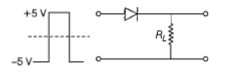then the output across RL will be
(a)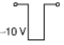(b)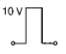(c)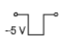(d)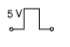Concept Questions :-

Logic gates
High Yielding Test Series + Question Bank - NEET 2020

Difficulty Level:

In the given figure, a diode D is connected to an external resistance R = 100 Ω  and an e.m.f of 3.5 V. If the barrier potential developed across the diode is 0.5 V, the current in the circuit will be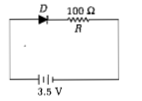1. 30mA

2. 40mA

3. 20mA

4. 35mA

Concept Questions :-

PN junction
High Yielding Test Series + Question Bank - NEET 2020

Difficulty Level:

The input signal given to a CE amplifier having a voltage gain of 150 is Vi=2cos(15t+π/3). The corresponding output signal will be

(a)300cos(15t+π/3)

(b)75cos(15t+2π/3)

(c)2cos(15t+5π/3)

(d)300cos(15t+4π/3)

High Yielding Test Series + Question Bank - NEET 2020

Difficulty Level:

The given graph represents V-I characteristic for a semiconductor device. Which of the following statement is correct?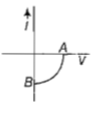(a) It is V-I characteristic for solar cell where point A represents open circuit voltage and point B short circuit current

(b) It is for a solar cell and points A and B represent open circuit voltage and current, respectively

(c) It is for a photodiode and points A and B represent open circuit voltage and current, respectively

(d) It is for a LED and points A and B represents open circuit voltage and short circuit current respectively

Concept Questions :-

Applications of PN junction
High Yielding Test Series + Question Bank - NEET 2020

Difficulty Level:

The barrier potential of a p-n junction depends on

(i)type of semiconductor material

(ii)amount of doping

(iii)temperature

Which one of the following is correct

1. (i) and (ii)only

2. (ii) only

3. (ii) and (iii)only

4. (i),(ii) and (iii)

Concept Questions :-

PN junction
High Yielding Test Series + Question Bank - NEET 2020

Difficulty Level:

In a n-type semiconductor, which of the following statement is true ?

(a) Electrons are majority carriers and trivalent atoms are dopants

(b) Electrons are minority carriers and pentavalent atoms are dopants

(c) Holes are minority carriers and pentavalent atoms are dopants

(d) Holes are majority carriers and trivalent atoms are dopants

Concept Questions :-

Types of semiconductors
High Yielding Test Series + Question Bank - NEET 2020

Difficulty Level:

The output (x) of logic circuit shown in figure will be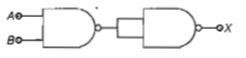(a) $X=\stackrel{=}{A}·\stackrel{=}{B}$

(b) $X=\overline{A.B}$

(c)$X=A·B$

(d)$X=\overline{A+B}$

Concept Questions :-

Logic gates
High Yielding Test Series + Question Bank - NEET 2020

Difficulty Level:

Two ideal diodes are connected to a battery as shown in the circuit. The current supplied by the battery is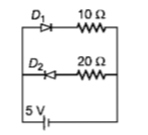(a)0.75A                              (b)zero

(c)0.25A                               (d)0.5A

Concept Questions :-

PN junction
High Yielding Test Series + Question Bank - NEET 2020

Difficulty Level:

In a CE transistor amplifier, the audio signal voltage across the collector resistance of 2  If the base resistance is 1 $k\Omega$ and the current amplification of the transistor is 100, the input signal voltage is

1. 0.1 V                                         2. 1.0 V

3. 1mV                                         4. 10mV

Concept Questions :-

Transistor